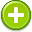#approximate 中文解釋 wordnet sense Collocation Usage
/əˈpräksəmit/,Font size:Close to the actual, but not completely accurate or exact,
1. Close to the actual, but not completely accurate or exact
• - the calculations are very approximate
Verb
1. Come close or be similar to something in quality, nature, or quantity
• - a leasing agreement approximating to ownership
• - reality can be approximated by computational techniques
2. Estimate or calculate (a quantity) fairly accurately
• - I had to approximate the weight of my horse

1. be close or similar; "Her results approximate my own"
2. not quite exact or correct; "the approximate time was 10 o'clock"; "a rough guess"; "a ballpark estimate"
3. estimate: judge tentatively or form an estimate of (quantities or time); "I estimate this chicken to weigh three pounds"
4. very close in resemblance; "sketched in an approximate likeness"; "a near likeness"
5. located close together; "with heads close together"; "approximate leaves grow together but are not united"
6. (approximately) (of quantities) imprecise but fairly close to correct; "lasted approximately an hour"; "in just about a minute"; "he's about 30 years old"; "I've had about all I can stand"; "we meet about once a month"; "some forty people came"; "weighs around a hundred pounds"; "roughly \$3,000" ...
7. (approximation) estimate: an approximate calculation of quantity or degree or worth; "an estimate of what it would cost"; "a rough idea how long it would take"
8. (approximation) the quality of coming near to identity (especially close in quantity)
9. (approximation) an imprecise or incomplete account; "newspapers gave only an approximation of the actual events"
10. An approximation is an inexact representation of something that is still close enough to be useful. Although approximation is most often applied to numbers, it is also frequently applied to such things as mathematical functions, shapes, and physical laws.
11. To carry or advance near; to cause to approach; To come near to; to approach; To estimate; Approaching; proximate; nearly resembling; Near correctness; nearly exact; not perfectly accurate
12. (approximately) imprecise but close to in quantity or amount
13. (2. approximately) پاس پاس ۔ قریب ۔ اک ۔ عن قریب ۔ قریب کر کے
14. ("Approximately") A tilde before a number used to mean "approximately". Example: "Jesus was born ~100 years ago."
15. (Approximately) normal distributions occur in many situations, as a result of the central limit theorem. When there is reason to suspect the presence of a large number of small effects acting additively and independently, it is reasonable to assume that observations will be normal. ...
16. (Approximates) The approximate tote odds on offer before the final pool is closed.
17. (Approximates) The TAB prices horses are showing before a race begins.
18. approximation is a mathematical quantity that estimates a desired quantity.
19. (Approximation) One small step, in a series of progressive steps that lead to the target behaviour.
20. (approximation) A process for generating estimated functional values at arbitrary locations beginning with a set of known functional values. At the known values, the approximated values need not equal the known values. This distinguishes an approximation from an interpolation.
21. The air is even less restricted than a fricative, meaning the tongue is only shaping the mouth cavity to produce a sound. English /l/, /r/, /w/, and /y/ are approximates. ...
22. adj. almost correct or exact but not completely so
23. A level of accuracy that is inexact or incorrect. Unless a Tolerance is explicitly indicated, "approximate" can mean anything from "as accurate as possible with professional application of normal measuring technology" to "wild-ass-guess from pacing the area". ...
24. This matching rule determines whether a presented value is approximately the same as a NDS stored value. The meaning of approximate must be determined by individual implementations of each syntax (only accessible using NWDSSearch()with the FTOK_APPROXIMATEtoken).
25. to bring edges of tissue together in direct apposition.